## Shape Functions and Basis Functions

In scientific computing the main aim of the tesselation is to provide a number of pairwise disjoint sets. Shape functions are transformed to single archetypical elements of the tesselation and basis functions for the function space are obtained. Each of these basis functions is non-zero on the given set (local support) whereas it is zero otherwise. The shape of these functions is typically the same for all sets of the tesselation.

Applying this method on all elements of the tesselation, a set of basis functions can be found based on the underlying tesselation of the simulation domain. In the following the construction of base functions is performed in detail for linearly bounded triangular elements. It is assumed that the domain is divided into subdomains of the same geometrical archetype, namely triangles. In this case it is possible to use standard functions which are based on the geometrical archetype of the elements. The single elements are transformed into the the standard element in order to evaluate the function value. For a triangle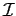a sensible choice are local linear shape functions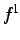as basis functions is (Fig. 2.2):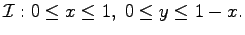(2.7)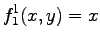(2.8)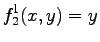(2.9)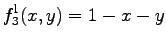(2.10)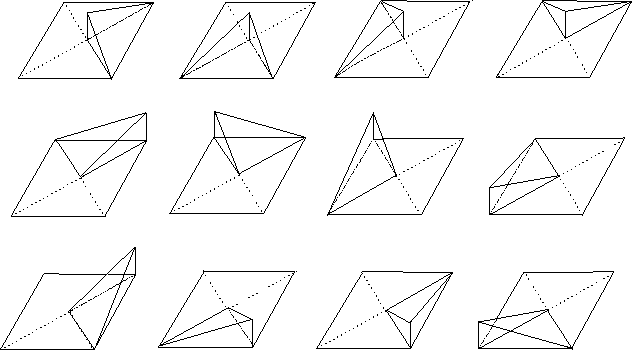It can be easily seen, that each function yields unity in one corner point and zero in all other corner points. This implies that the weighting coefficients of the single functions have the value of the function when evaluated the respective corner point.

These functions are applied to the standard triangle and this triangle is back-transformed in its original position. Of course, such a transformation can be applied on many different archetypes of elements and also the local shape functions can be altered. From this point of view the shape of these functions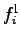can be chosen arbitrarily.

For the triangle example, the three shape functions are transformed to each triangular element. One obtains a function space with linear basis functions defined on local elements of the tesselation. However, it has to be mentioned that for such a function space even very essential properties such as continuity are not given.

In order to guarantee continuity it is necessary to use a subspace of the original function space defined by the local shape functions. The main aim is to search for a subspace in which the base function and therewith all functions are continuous. To form such a function space, some weighting coefficients have to be coupled in a predefined way. In this case it turns out that the collocation of functions and points as described above is convenient, because in the corner points, where several triangles intersect, the function values have to be identical in all triangles. For this reason the function space is restricted so that each shape function which is collocated with the same point has the same weighting factor. Of course, the coupling of the weighting factors restricts the possible functions of the function space and forms a subspace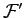of the initial space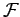. The basis functions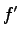of the newly defined subspaceof the original base space can be written in the following manner, where the points of the intersection of the triangles are denoted as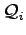.(2.11)

If the values of the shape functions in the collocation points are identically unity, the definition ofcan be simplified to (see Fig. 2. 3 )(2.12)

The resulting functions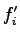have a different local support than the base functions of. These functions are defined within a neighborhood of the respective intersection point as can be seen in Fig. 2.3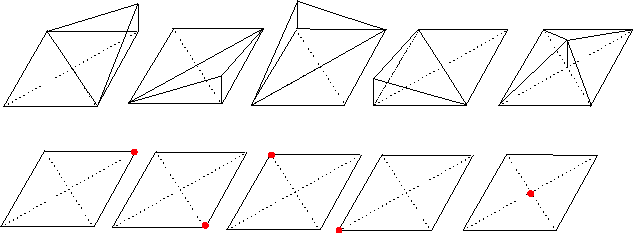In some cases it is favorable to use more shape functions on a base element. An example could be the use of third order shape functions which form the function space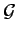. Such a function space can be based on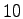basis functions, where three are collocated with the corner points of the triangle,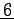are collocated with edge points, and one is collocated with a point the interior of the triangle.

It can be seen that the function spaceis a subspace of the function space. In analogy to the space, the property of continuity is not given in this case, because each function is based on a single triangle. In this case, however, the fitting of the functions is not restricted to a single point. While it is clear that continuity is given on the edge between two triangles, where the two end points of the edge are fit to the same value, this does not hold true for higher-order polynomial shape functions. For this reason, it is necessary to guarantee that continuity is preserved on the edge. Moreover, it can be stated that if more shape functions are available more restrictions on continuity as well as smoothness can be imposed by reducing the number of base functions.

Again, the collocation of functions and points on the triangle implies an easy fitting of neighboring triangles. In this case the collocation fitting is not only performed on the corner points of the triangle but also on two points of each edge. From this point of view it is clear that even though the use of collocation of points and shape functions is not coercive, other local shape functions imply further equations in the final equation system and, therefore, an unnecessary use of memory and computation time.

In analogy to the measures which have been taken to derive the global shape functions of the local linear shape functions the third order local shape functions yield the global shape functions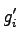of the function space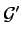by(2.13)

It has to be mentioned that in this case the collocation points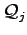are the corner points, points on the edges and points in the interior of the triangles. The functions which are collocated with the interior of the triangle have their local support only on the respective triangle. The functions which are collocated with an edge point of a triangle have their local support on both triangles which are supersets of the common edge. As already mentioned, the functions which are collocated with the corner points of the triangle have their local support on all triangles which cover the respective point.

It should be noted that the method which has been shown for triangles can also be used on many other archetypes such as tetrahedra, squares, prisms, and pyramides as long as a transformation into a standard element and local element functions are given.

Michael 2008-01-16